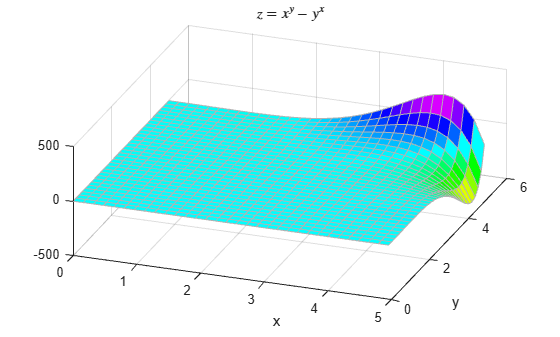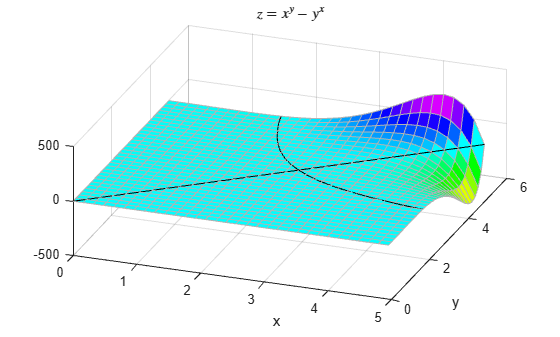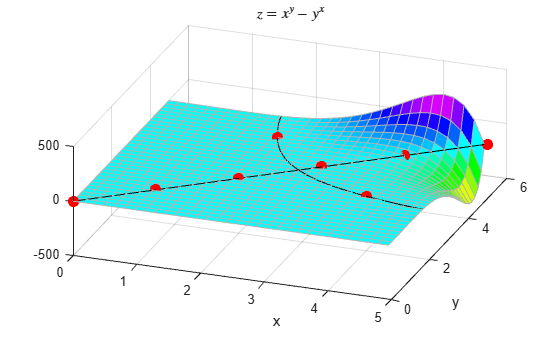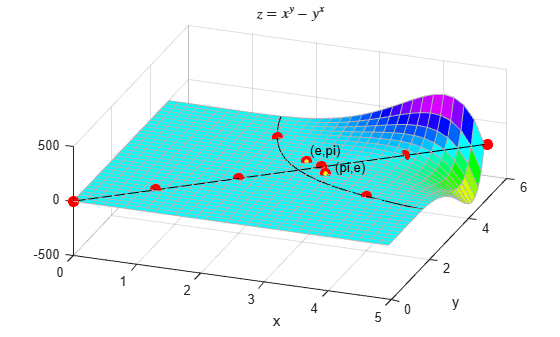# Graphical Comparison of Exponential Functions

This example shows an interesting graphical approach for discovering whether ${\mathrm{e}}^{\pi }$ is greater than ${\pi }^{\mathit{e}}$.

The question is: which is greater, ${\mathrm{e}}^{\pi }$ or ${\pi }^{\mathit{e}}$? The easy way to find out is to type it directly at the MATLAB® command prompt. But another way to analyze the situation is to ask a more general question: what is the shape of the function $\mathit{z}\left(\mathit{x},\mathit{y}\right)={\mathit{x}}^{\mathit{y}}-{\mathit{y}}^{\mathit{x}}$?

Here is a plot of $\mathit{z}$.

```% Define the mesh x = 0:0.16:5; y = 0:0.16:5; [xx,yy] = meshgrid(x,y); % The plot zz = xx.^yy-yy.^xx; h = surf(x,y,zz); h.EdgeColor = [0.7 0.7 0.7]; view(20,50); colormap(hsv); title('\$z = x^y-y^x\$','Interpreter','latex') xlabel('x') ylabel('y') hold on```The solution of the equation ${\mathit{x}}^{\mathit{y}}-{\mathit{y}}^{\mathit{x}}=0$ has a very interesting shape, and our original question is not easily solved by inspection. Here is a plot of the xy values that yield $\mathit{z}=0$.

```c = contourc(x,y,zz,[0 0]); list1Len = c(2,1); xContour = [c(1,2:1+list1Len) NaN c(1,3+list1Len:size(c,2))]; yContour = [c(2,2:1+list1Len) NaN c(2,3+list1Len:size(c,2))]; % Note that the NAN above prevents the end of the first contour line from being % connected to the beginning of the second line line(xContour,yContour,'Color','k');```Some combinations of x and y along the black curve are integers. This next plot is of the integer solutions to the equation ${\mathit{x}}^{\mathit{y}}-{\mathit{y}}^{\mathit{x}}=0$. Notice that ${2}^{4}={4}^{2}$ is the only integer solution where $\mathit{x}\ne \mathit{y}$.

`plot([0:5 2 4],[0:5 4 2],'r.','MarkerSize',25);`Finally, plot the points $\left(\pi ,\mathit{e}\right)$ and $\left(\mathit{e},\pi \right)$ on the surface. The result shows that ${\mathrm{e}}^{\pi }$ is indeed larger than ${\pi }^{\mathit{e}}$ (though not by much).

```e = exp(1); plot([e pi],[pi e],'r.','MarkerSize',25); plot([e pi],[pi e],'y.','MarkerSize',10); text(e,3.3,'(e,pi)','Color','k', ... 'HorizontalAlignment','left','VerticalAlignment','bottom'); text(3.3,e,'(pi,e)','Color','k','HorizontalAlignment','left',... 'VerticalAlignment','bottom'); hold off;```Verify the results.

```e = exp(1); e^pi```
```ans = 23.1407 ```
`pi^e`
```ans = 22.4592 ```